Back to Contents

# Sorting Things

Lists often need to be in sorted order. How might we write a function to sort a list of integers? Well, a list with zero elements is already sorted. If we do not have an empty list, we must have a head and a tail. What can we do with those? Well, we can sort the tail by a recursive call to our `sort` function. So, now we have the head, and an already sorted list. Now, we just need to write a function to insert the head in an already sorted list. We have reduced the problem to an easier one.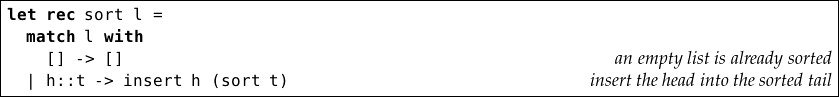Now we just need to write the `insert` function. This takes an element and an already-sorted list, and returns the list with the element inserted in the right place: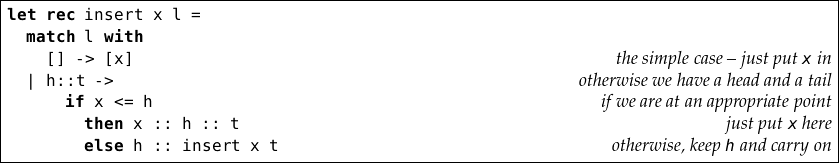Consider the evaluation of `insert 3 [1; 1; 2; 3; 5; 9]`: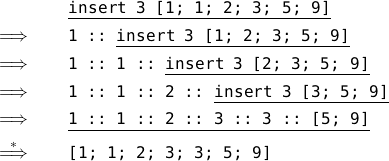Here is the whole evaluation of `sort [53; 9; 2; 6; 19]`. We have missed out the detail of each `insert` operation.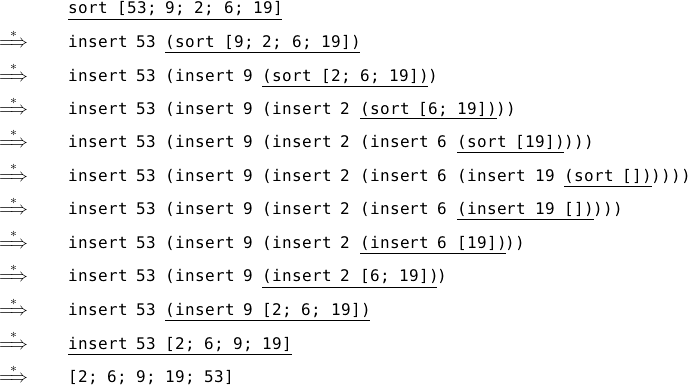Here’s the full program, known as insertion sort: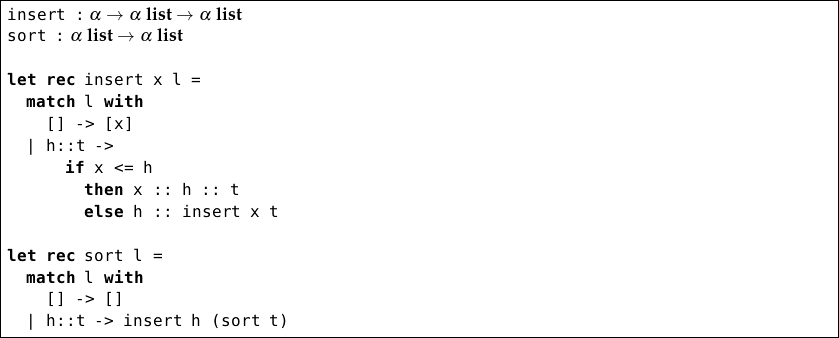Notice that the type α list α list rather than int list int list. This is because OCaml’s comparison functions like `<=` (used inside `insert`) work for types other than int. For example, OCaml knows how to compare characters in alphabetical order: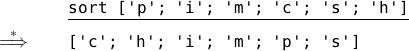How long does our sorting function take to run if the list to be sorted has n elements? Under the assumption that our argument list is arbitrarily ordered rather than sorted, each `insert` operation takes time proportional to n (the element might need to be inserted anywhere). We must run the `insert` function as many times as there are elements so, adding these all up, the `sort` function takes time proportional to n2. You might argue that the first `insert` operations only have to work with a very small list, and that this fact should make the time less that n2. Can you see why that is not true? What happens if the list is sorted already?

A more efficient algorithm can be found by considering a basic operation a little more complex than `insert`, but which still operates in time proportional to the length of the argument list. Such a function is `merge`, which takes two already sorted lists, and returns a single sorted list: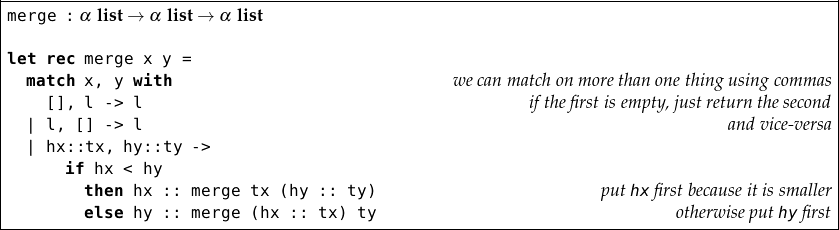When `x` and `y` are both the empty list, the first case is picked because `l` matches the empty list. Here is how `merge` proceeds: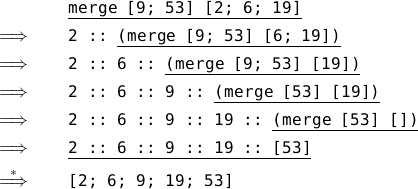So merge can take two sorted lists, and produce a longer, sorted list, containing all the elements from both lists. So, how can we use this to sort a list from scratch? Well, we can use `length`, `take`, and `drop` from the previous chapter to split the list into two halves. Now, we must use a recursive call to sort each half, and then we can merge them. This is known as merge sort.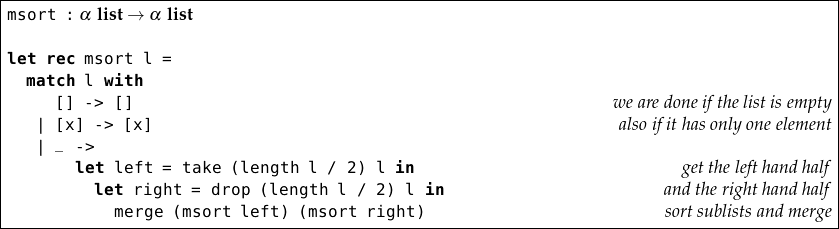The case for the single element is required because, if we split it into two halves, of length one and zero, the recursion would not end – we would not have reduced the size of the problem.

How does `msort` work? Consider the evaluation of `msort` on the list `[53; 9; 2; 6; 19]`. We will skip the evaluation of the `merge`, `drop`, `take`, and `length` functions, concentrating just on `msort`: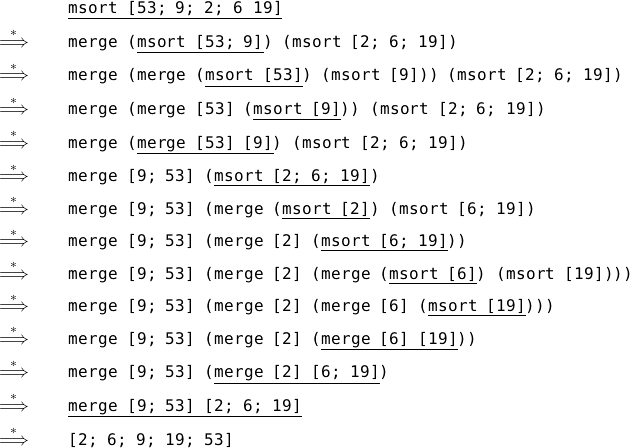From now on we will not be showing these full evaluations all the time – but when you are unsure of how or why a function works, you can always write them out on paper yourself.

## How long does it take?

How long does merge sort take to run? We can visualize it with the following diagram, in which we have chosen a list of length eight (a power of two) for convenience.

`[6; 4; 5; 7; 2; 5; 3; 4]`
`[6; 4; 5; 7][2; 5; 3; 4]`
`[6; 4][5; 7][2; 5][3; 4]`
``
`[4; 6][5; 7][2; 5][3; 4]`
`[4; 5; 6; 7][2; 3; 4; 5]`
`[2; 3; 4; 4; 5; 5; 6; 7]`

In the top half of the diagram, the lists are being taken apart using `take` and `drop`, until they are small enough to already be sorted. In the bottom half, they are being merged back together.

How long does each row take? For the top half: to split a list into two halves takes time proportional to the length of the list. On the first line, we do this once on a list of length eight. On the second line, we do it twice on lists of length four, and so on. So each line takes the same time overall. For the bottom half, we have another function which takes time proportional to the length of its argument – `merge` – so each line in the bottom half takes time proportional to the length too.

So, how many lines do we have? Well, in the top half we have roughly log2n, and the same for the bottom half. So, the total work done is 2 × log2n × n, which is proportional to nlog2n.

## Questions

1. In `msort`, we calculate the value of the expression `length l / 2` twice. Modify `msort` to remove this inefficiency.

2. We know that `take` and `drop` can fail if called with incorrect arguments. Show that this is never the case in `msort`.

3. Write a version of insertion sort which sorts the argument list into reverse order.

4. Write a function to detect if a list is already in sorted order.

5. We mentioned that the comparison functions like  `<`  work for many OCaml types. Can you determine, by experimentation, how they work for lists? For example, what is the result of `[1; 2] < [2; 3]`? What happens when we sort the following list of type char list list? Why?

`[[’o’; ’n’; ’e’]; [’t’; ’w’; ’o’]; [’t’; ’h’; ’r’; ’e’; ’e’]]`

6. Combine the `sort` and `insert` functions into a single `sort` function.